Anzeige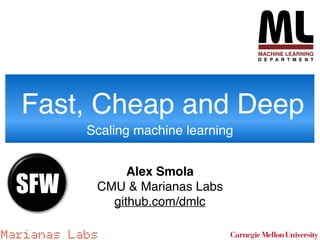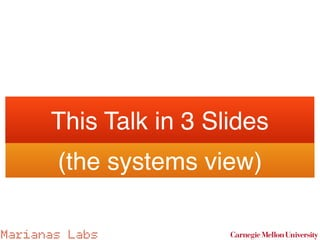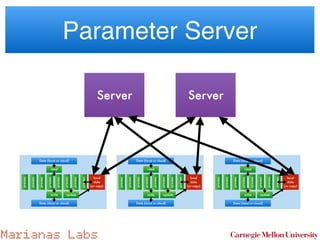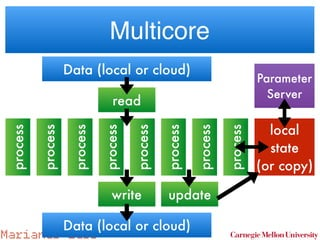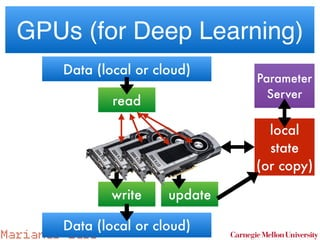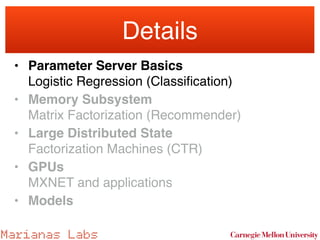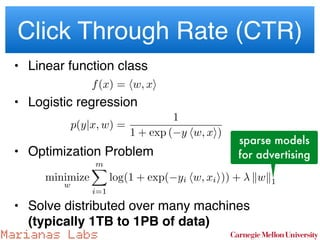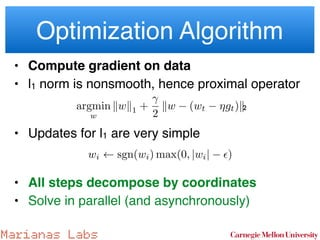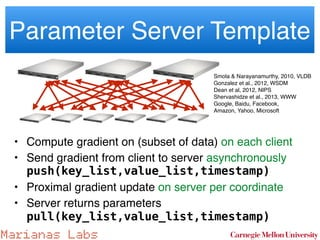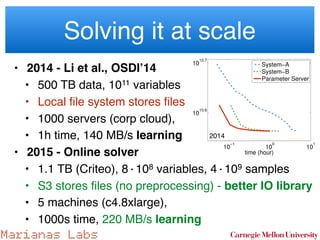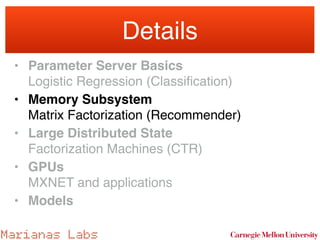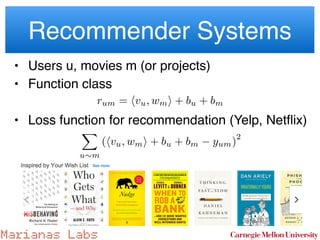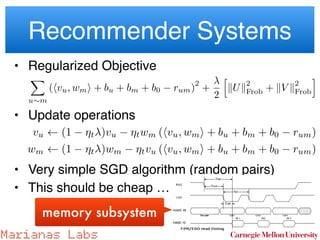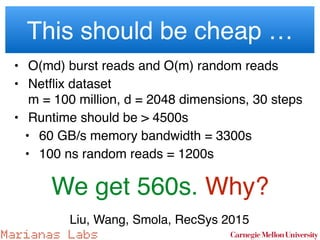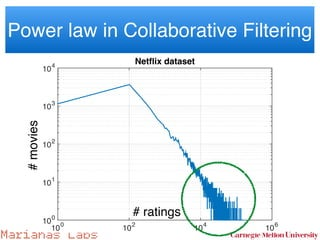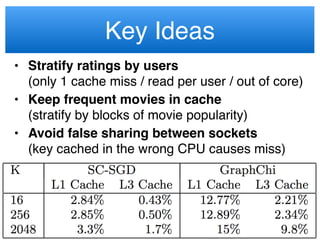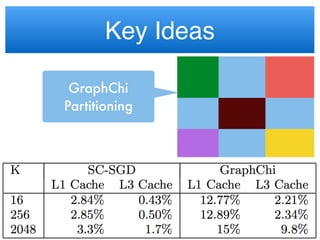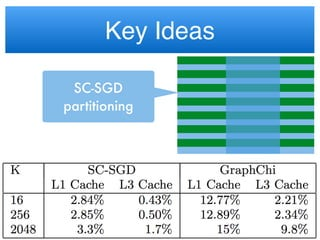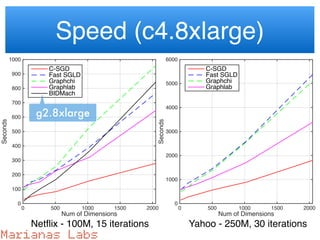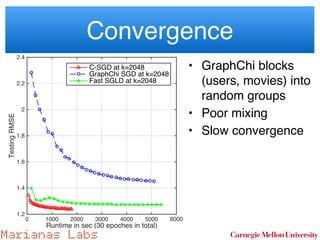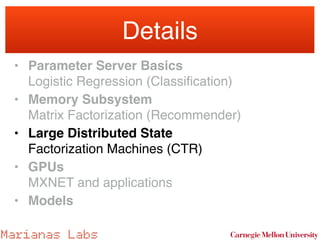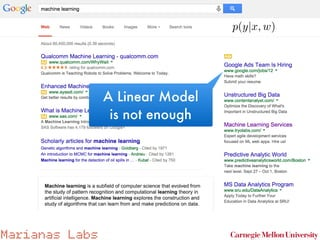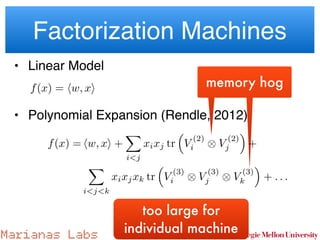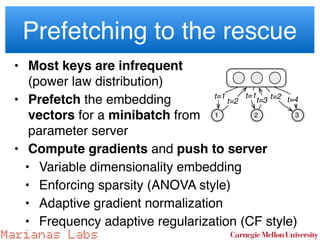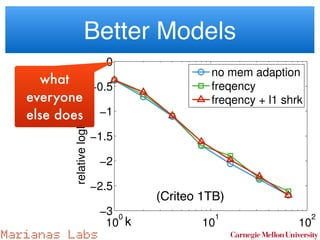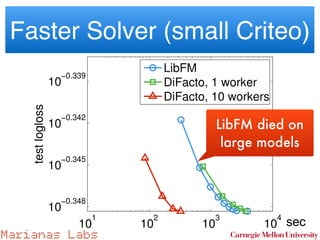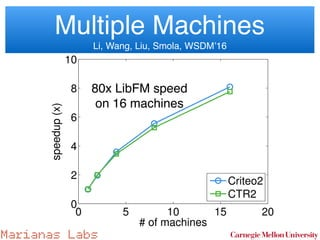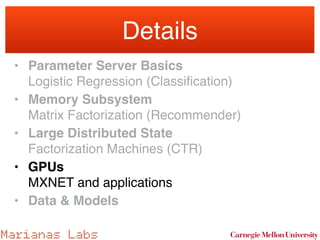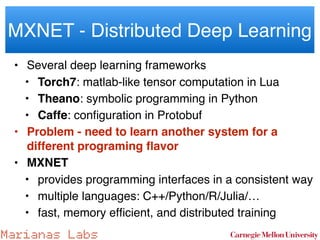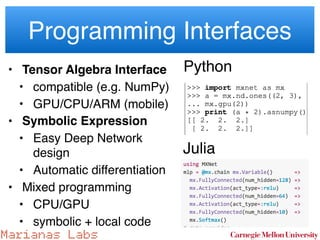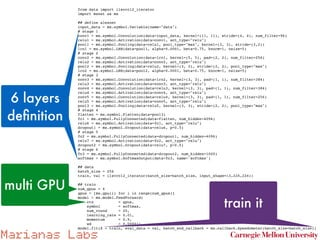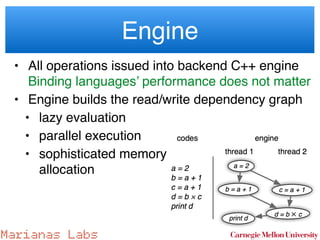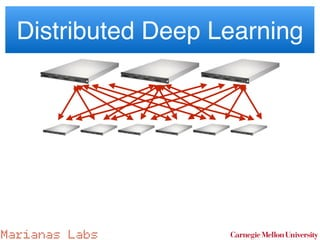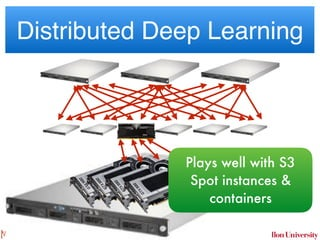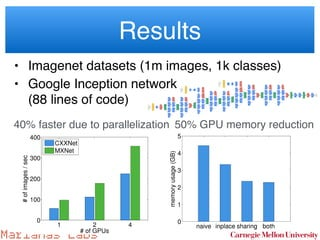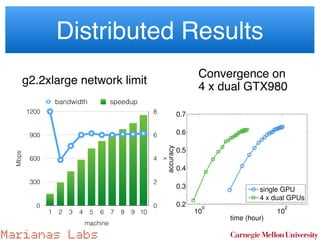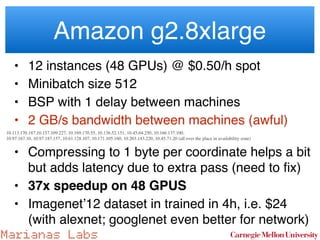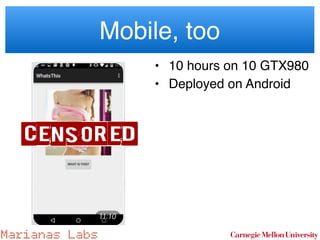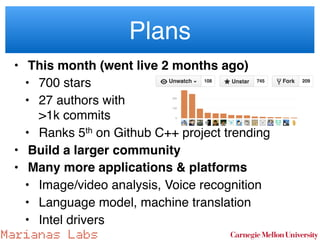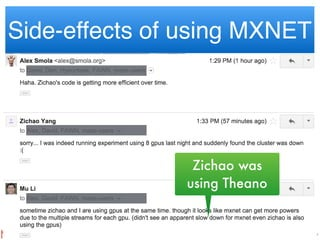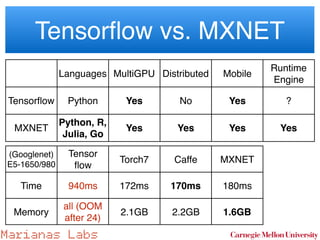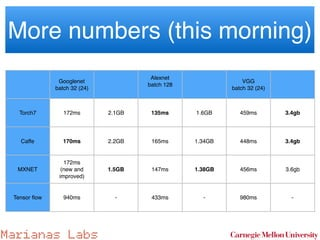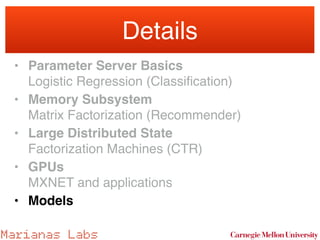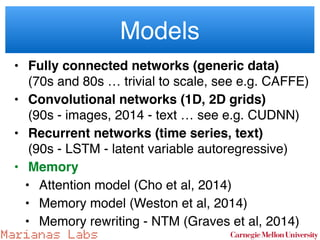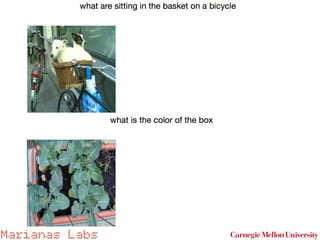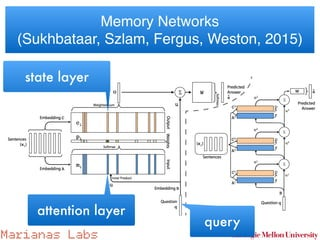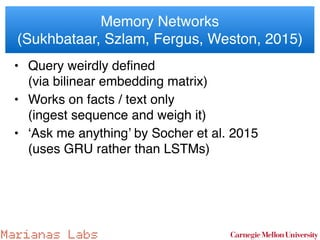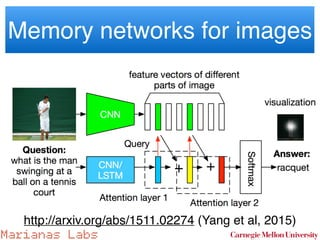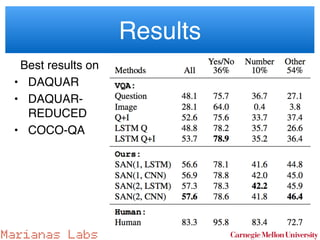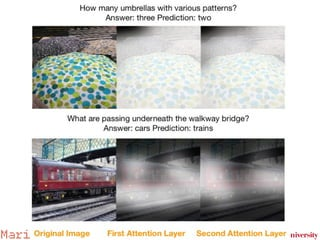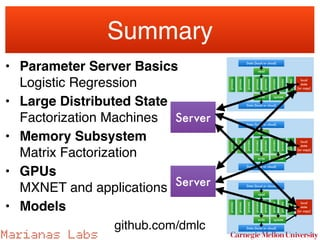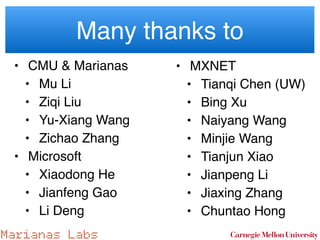1 von 54
Anzeige

### Alex Smola, Professor in the Machine Learning Department, Carnegie Mellon University at MLconf SF - 11/13/15

1. Marianas Labs Alex Smola CMU & Marianas Labs github.com/dmlc Fast, Cheap and Deep Scaling machine learning SFW
2. Marianas Labs This Talk in 3 Slides (the systems view)
3. Marianas Labs Parameter Server Data (local or cloud) read process process process process process process process process write Data (local or cloud) local state (or copy) update Server Data (local or cloud) read process process process process process process process process write Data (local or cloud) local state (or copy) update Data (local or cloud) read process process process process process process process process write Data (local or cloud) local state (or copy) update Server
4. Marianas Labs Data (local or cloud) read process process process process process process process process write Data (local or cloud) local state (or copy) update Parameter Server Multicore
5. Marianas Labs Data (local or cloud) read write Data (local or cloud) local state (or copy) update Parameter Server GPUs (for Deep Learning)
6. Marianas Labs Details • Parameter Server Basics  Logistic Regression (Classiﬁcation) • Memory Subsystem  Matrix Factorization (Recommender) • Large Distributed State  Factorization Machines (CTR) • GPUs  MXNET and applications • Models
7. Marianas Labs Estimate Click Through Rate p(click|{z} =:y | ad, query | {z } =:x , w)
8. Marianas Labs sparse models for advertising Click Through Rate (CTR) • Linear function class • Logistic regression    • Optimization Problem • Solve distributed over many machines  (typically 1TB to 1PB of data) f(x) = hw, xi minimize w log p(f|X, Y ) minimize w mX i=1 log(1 + exp( yi hw, xii)) + kwk1 p(y|x, w) = 1 1 + exp ( y hw, xi)
9. Marianas Labs Optimization Algorithm • Compute gradient on data • l1 norm is nonsmooth, hence proximal operator    • Updates for l1 are very simple    • All steps decompose by coordinates • Solve in parallel (and asynchronously) argmin w kwk1 + 2 kw (wt ⌘gt)k wi sgn(wi) max(0, |wi| ✏) 2
10. Marianas Labs Parameter Server Template • Compute gradient on (subset of data) on each client • Send gradient from client to server asynchronously  push(key_list,value_list,timestamp) • Proximal gradient update on server per coordinate • Server returns parameters  pull(key_list,value_list,timestamp) Smola & Narayanamurthy, 2010, VLDB Gonzalez et al., 2012, WSDM Dean et al, 2012, NIPS Shervashidze et al., 2013, WWW Google, Baidu, Facebook,   Amazon, Yahoo, Microsoft
11. Marianas Labs w2Rp i=1 where the regularizer kwk1 has a desirable property to control the number of non-zero entries in the optimal solu- tion w⇤ , but its non-smooth property makes this objective function hard to be solved. We compared parameter server with two speciﬁc- purpose systems developed by an Internet company. For privacy purpose, we name them System-A, and System- B respectively. The former uses an variant of the well- known L-BFGS [21, 5], while the latter runs an variant of block proximal gradient method , which updates a block of parameters at each iteration according to the ﬁrst- order and diagonal second-order gradients. Both systems use sequential consistency model, but are well optimized in both computation and communication. We re-implemented the algorithm used by System-B on parameter server. Besides, we made two modiﬁcations. One is that we relax the consistency model to bounded delay. The other one is a KKT ﬁlter to avoid sending gra- dients which may do not affect the parameters. Speciﬁcally, let gk be the global (ﬁrst-order) gradient on feature k at iteration t. Then, the according parameter wk will not be changed at this iteration if wk = 0 and  gk  due to the update rule. Therefore it is not necessary for workers to send gk at this iteration. But a worker does not know the global gk without communica- tion, instead, we let a worker i approximate gk based on its local gradient gi k by ˜gk = ckgi k/ci k, where ck is the each one has 16 cores, 192GB memory, and are connec by 10GB Ethernet. For parameter server, we use 800 chines to form the worker group. Each worker cac around 1 billions of parameters. The rest 200 mach make the server group, where each machine runs 10 ( tual) server nodes. 10 −1 10 0 10 1 10 10.6 10 10.7 time (hour) objectivevalue System−A System−B Parameter Server Figure 8: Convergence results of sparse logistic reg sion, the goal is to achieve small objective value us less time. We run these three systems to achieve the same ob tive value, the less time used the better. Both system and parameter server use 500 blocks. In addition, par eter server ﬁx ⌧ = 4 for the bounded delay, which me each worker can parallel executes 4 blocks. Solving it at scale • 2014 - Li et al., OSDI’14 • 500 TB data, 1011 variables • Local ﬁle system stores ﬁles • 1000 servers (corp cloud), • 1h time, 140 MB/s learning • 2015 - Online solver • 1.1 TB (Criteo), 8·108 variables, 4·109 samples • S3 stores ﬁles (no preprocessing) - better IO library • 5 machines (c4.8xlarge), • 1000s time, 220 MB/s learning 2014
12. Marianas Labs Details • Parameter Server Basics  Logistic Regression (Classiﬁcation) • Memory Subsystem  Matrix Factorization (Recommender) • Large Distributed State  Factorization Machines (CTR) • GPUs  MXNET and applications • Models
13. Marianas Labs Recommender Systems • Users u, movies m (or projects) • Function class • Loss function for recommendation (Yelp, Netﬂix)    rum = hvu, wmi + bu + bm X u⇠m (hvu, wmi + bu + bm yum) 2
14. Marianas Labs Recommender Systems • Regularized Objective    • Update operations • Very simple SGD algorithm (random pairs) • This should be cheap … X u⇠m (hvu, wmi + bu + bm + b0 rum) 2 + 2 h kUk 2 Frob + kV k 2 Frob i vu (1 ⌘t )vu ⌘twm (hvu, wmi + bu + bm + b0 rum) wm (1 ⌘t )wm ⌘tvu (hvu, wmi + bu + bm + b0 rum) memory subsystem
15. Marianas Labs This should be cheap … • O(md) burst reads and O(m) random reads • Netﬂix dataset   m = 100 million, d = 2048 dimensions, 30 steps • Runtime should be > 4500s • 60 GB/s memory bandwidth = 3300s • 100 ns random reads = 1200s  We get 560s. Why? Liu, Wang, Smola, RecSys 2015
16. Marianas Labs Power law in Collaborative Filtering # of ratings 10 0 10 2 10 4 10 6 Movieitemcounts 10 0 101 102 10 3 104 Netflix dataset # ratings #movies
17. Marianas Labs Key Ideas • Stratify ratings by users   (only 1 cache miss / read per user / out of core) • Keep frequent movies in cache  (stratify by blocks of movie popularity) • Avoid false sharing between sockets  (key cached in the wrong CPU causes miss)
18. Marianas Labs Key Ideas GraphChi Partitioning
19. Marianas Labs Key Ideas SC-SGD partitioning
20. Marianas Labs Speed (c4.8xlarge) Num of Dimensions 0 500 1000 1500 2000 Seconds 0 100 200 300 400 500 600 700 800 900 1000 C-SGD Fast SGLD Graphchi Graphlab BIDMach Num of Dimensions 0 500 1000 1500 2000 Seconds 0 1000 2000 3000 4000 5000 6000 C-SGD Fast SGLD Graphchi Graphlab Netﬂix - 100M, 15 iterations Yahoo - 250M, 30 iterations g2.8xlarge
21. Marianas Labs Convergence • GraphChi blocks (users, movies) into random groups • Poor mixing • Slow convergence Runtime in sec (30 epoches in total) 0 1000 2000 3000 4000 5000 6000 TestingRMSE 1.2 1.4 1.6 1.8 2 2.2 2.4 C-SGD at k=2048 GraphChi SGD at k=2048 Fast SGLD at k=2048
22. Marianas Labs Details • Parameter Server Basics  Logistic Regression (Classiﬁcation) • Memory Subsystem  Matrix Factorization (Recommender) • Large Distributed State  Factorization Machines (CTR) • GPUs  MXNET and applications • Models
23. Marianas Labs A Linear Model is not enough p(y|x, w)
24. Marianas Labs Factorization Machines • Linear Model    • Polynomial Expansion (Rendle, 2012) memory hogmemory hog too large for individual machine f(x) = hw, xi f(x) = hw, xi + X i<j xixj tr ⇣ V (2) i ⌦ V (2) j ⌘ + X i<j<k xixjxk tr ⇣ V (3) i ⌦ V (3) j ⌦ V (3) k ⌘ + . . .
25. Marianas Labs Prefetching to the rescue • Most keys are infrequent  (power law distribution) • Prefetch the embedding  vectors for a minibatch from  parameter server • Compute gradients and push to server • Variable dimensionality embedding • Enforcing sparsity (ANOVA style) • Adaptive gradient normalization • Frequency adaptive regularization (CF style) a minibatch. The consequence of this is associated with frequent features are less The penalty can be presented as X i ⇥ iw2 i + µi kVik2 2 ⇤ with i / µi. (11) namic Regularization) Assume that we solve machines problem with the following updates pdate setting: for each feature i, 6= 0 for some (xj, yj) 2 B} (12) X (xj ,yj )2B @wi l(f(xj), yj) ⌘t wiIi (13) X (xj ,yj )2B @Vi l(f(xj), yj) ⌘tµViIi (14) the minibatch and b the according size, and er or not feature i appears in this minibatch. gularization is given by 1 2 3 server nodes: worker nodes: t=1 t=1 t=2 t=2 t=3 t=4 Figure 1: An illustration of asynchronous SGD. Wo W1 and W2 ﬁrst pull weights from the servers at tim Next W1 pushes the gradient back and therefore incr the timestamp at the servers. Worker W3 pulls the we immediately after that. Then W2 and W3 push the grad back. The delays for W1, W2, and W3 are 0, 1, and 1. data preprocessing. In this paper, we consider asynchro stochastic gradient descent (SGD)  for optimization. 4.1 Asynchronous Stochastic Gradient Des
26. Marianas Labs Better Models10 0 10 1 10 2 300 (b) Runtim 10 0 10 1 10 2 −3 −2.5 −2 −1.5 −1 −0.5 0 dimesion (k) relativelogloss(%) no mem adaption freqency freqency + l1 shrk (Criteo 1TB) k what everyone else does
27. Marianas Labs Faster Solver (small Criteo) 10 1 10 2 10 3 10 4 10 −0.348 10 −0.345 10 −0.342 10 −0.339 time (sec) testlogloss LibFM DiFacto, 1 worker DiFacto, 10 workers sec LibFM died on large models
28. Marianas Labs 0 5 10 15 20 0 2 4 6 8 10 # of machines speedup(x) Criteo2 CTR2 Multiple Machines   Li, Wang, Liu, Smola, WSDM’16 80x LibFM speed  on 16 machines
29. Marianas Labs Details • Parameter Server Basics  Logistic Regression (Classiﬁcation) • Memory Subsystem  Matrix Factorization (Recommender) • Large Distributed State  Factorization Machines (CTR) • GPUs  MXNET and applications • Data & Models
30. Marianas Labs MXNET - Distributed Deep Learning • Several deep learning frameworks • Torch7: matlab-like tensor computation in Lua • Theano: symbolic programming in Python • Caffe: conﬁguration in Protobuf • Problem - need to learn another system for a different programing ﬂavor • MXNET • provides programming interfaces in a consistent way • multiple languages: C++/Python/R/Julia/… • fast, memory efﬁcient, and distributed training
31. Marianas Labs Programming Interfaces • Tensor Algebra Interface • compatible (e.g. NumPy) • GPU/CPU/ARM (mobile) • Symbolic Expression • Easy Deep Network design • Automatic differentiation • Mixed programming • CPU/GPU • symbolic + local code Python Julia
32. Marianas Labs from data import ilsvrc12_iterator import mxnet as mx ## define alexnet input_data = mx.symbol.Variable(name="data") # stage 1 conv1 = mx.symbol.Convolution(data=input_data, kernel=(11, 11), stride=(4, 4), num_filter=96) relu1 = mx.symbol.Activation(data=conv1, act_type="relu") pool1 = mx.symbol.Pooling(data=relu1, pool_type="max", kernel=(3, 3), stride=(2,2)) lrn1 = mx.symbol.LRN(data=pool1, alpha=0.0001, beta=0.75, knorm=1, nsize=5) # stage 2 conv2 = mx.symbol.Convolution(data=lrn1, kernel=(5, 5), pad=(2, 2), num_filter=256) relu2 = mx.symbol.Activation(data=conv2, act_type="relu") pool2 = mx.symbol.Pooling(data=relu2, kernel=(3, 3), stride=(2, 2), pool_type="max") lrn2 = mx.symbol.LRN(data=pool2, alpha=0.0001, beta=0.75, knorm=1, nsize=5) # stage 3 conv3 = mx.symbol.Convolution(data=lrn2, kernel=(3, 3), pad=(1, 1), num_filter=384) relu3 = mx.symbol.Activation(data=conv3, act_type="relu") conv4 = mx.symbol.Convolution(data=relu3, kernel=(3, 3), pad=(1, 1), num_filter=384) relu4 = mx.symbol.Activation(data=conv4, act_type="relu") conv5 = mx.symbol.Convolution(data=relu4, kernel=(3, 3), pad=(1, 1), num_filter=256) relu5 = mx.symbol.Activation(data=conv5, act_type="relu") pool3 = mx.symbol.Pooling(data=relu5, kernel=(3, 3), stride=(2, 2), pool_type="max") # stage 4 flatten = mx.symbol.Flatten(data=pool3) fc1 = mx.symbol.FullyConnected(data=flatten, num_hidden=4096) relu6 = mx.symbol.Activation(data=fc1, act_type="relu") dropout1 = mx.symbol.Dropout(data=relu6, p=0.5) # stage 5 fc2 = mx.symbol.FullyConnected(data=dropout1, num_hidden=4096) relu7 = mx.symbol.Activation(data=fc2, act_type="relu") dropout2 = mx.symbol.Dropout(data=relu7, p=0.5) # stage 6 fc3 = mx.symbol.FullyConnected(data=dropout2, num_hidden=1000) softmax = mx.symbol.SoftmaxOutput(data=fc3, name='softmax') ## data batch_size = 256 train, val = ilsvrc12_iterator(batch_size=batch_size, input_shape=(3,224,224)) ## train num_gpus = 4 gpus = [mx.gpu(i) for i in range(num_gpus)] model = mx.model.FeedForward( ctx = gpus, symbol = softmax, num_round = 20, learning_rate = 0.01, momentum = 0.9, wd = 0.00001) model.fit(X = train, eval_data = val, batch_end_callback = mx.callback.Speedometer(batch_size=batch_size)) 6 layers deﬁnition 6 layers deﬁnition multi GPUmulti GPU train ittrain it
33. Marianas Labs Engine • All operations issued into backend C++ engine  Binding languages’ performance does not matter • Engine builds the read/write dependency graph • lazy evaluation • parallel execution • sophisticated memory  allocation
34. Marianas Labs Distributed Deep Learning
35. Marianas Labs Distributed Deep Learning Plays well with S3  Spot instances & containers
36. Marianas Labs • Imagenet datasets (1m images, 1k classes) • Google Inception network   (88 lines of code) Results 40% faster due to parallelization 1 2 4 0 100 200 300 400 #ofimages/sec # of GPUs CXXNet MXNet naive inplace sharing both 0 1 2 3 4 5 memoryusage(GB) 50% GPU memory reduction
37. Marianas Labs Distributed Results x 0 2 4 6 8 Mbps 0 300 600 900 1200 machine 1 2 3 4 5 6 7 8 9 10 bandwidth speedup g2.2xlarge network limit 10 0 10 2 0.2 0.3 0.4 0.5 0.6 0.7 time (hour) accuracy single GPU 4 x dual GPUs Convergence on 4 x dual GTX980
38. Marianas Labs Amazon g2.8xlarge • 12 instances (48 GPUs) @ $0.50/h spot • Minibatch size 512 • BSP with 1 delay between machines • 2 GB/s bandwidth between machines (awful) 10.113.170.187,10.157.109.227, 10.169.170.55, 10.136.52.151, 10.45.64.250, 10.166.137.100, 10.97.167.10, 10.97.187.157, 10.61.128.107, 10.171.105.160, 10.203.143.220, 10.45.71.20 (all over the place in availability zone) • Compressing to 1 byte per coordinate helps a bit but adds latency due to extra pass (need to ﬁx) • 37x speedup on 48 GPUS • Imagenet’12 dataset in trained in 4h, i.e.$24  (with alexnet; googlenet even better for network)
39. Marianas Labs Mobile, too • 10 hours on 10 GTX980 • Deployed on Android
40. Marianas Labs Plans • This month (went live 2 months ago) • 700 stars • 27 authors with  >1k commits • Ranks 5th on Github C++ project trending • Build a larger community • Many more applications & platforms • Image/video analysis, Voice recognition • Language model, machine translation • Intel drivers
41. Marianas Labs Side-effects of using MXNET Zichao was using Theano
42. Marianas Labs Tensorﬂow vs. MXNET Languages MultiGPU Distributed Mobile Runtime Engine Tensorﬂow Python Yes No Yes ? MXNET Python, R, Julia, Go Yes Yes Yes Yes (Googlenet) E5-1650/980 Tensor ﬂow Torch7 Caffe MXNET Time 940ms 172ms 170ms 180ms Memory all (OOM after 24) 2.1GB 2.2GB 1.6GB
43. Marianas Labs More numbers (this morning) Googlenet batch 32 (24) Alexnet  batch 128 VGG batch 32 (24) Torch7 172ms 2.1GB 135ms 1.6GB 459ms 3.4gb Caffe 170ms 2.2GB 165ms 1.34GB 448ms 3.4gb MXNET 172ms  (new and improved) 1.5GB 147ms 1.38GB 456ms 3.6gb Tensor ﬂow 940ms - 433ms - 980ms -
44. Marianas Labs Details • Parameter Server Basics  Logistic Regression (Classiﬁcation) • Memory Subsystem  Matrix Factorization (Recommender) • Large Distributed State  Factorization Machines (CTR) • GPUs  MXNET and applications • Models
45. Marianas Labs Models • Fully connected networks (generic data)  (70s and 80s … trivial to scale, see e.g. CAFFE) • Convolutional networks (1D, 2D grids)  (90s - images, 2014 - text … see e.g. CUDNN) • Recurrent networks (time series, text)  (90s - LSTM - latent variable autoregressive) • Memory • Attention model (Cho et al, 2014) • Memory model (Weston et al, 2014) • Memory rewriting - NTM (Graves et al, 2014)
46. Marianas Labs
47. Marianas Labs Memory Networks  (Sukhbataar, Szlam, Fergus, Weston, 2015) attention layer state layer query
48. Marianas Labs Memory Networks  (Sukhbataar, Szlam, Fergus, Weston, 2015) • Query weirdly deﬁned  (via bilinear embedding matrix) • Works on facts / text only  (ingest sequence and weigh it) • ‘Ask me anything’ by Socher et al. 2015  (uses GRU rather than LSTMs)
49. Marianas Labs Memory networks for images http://arxiv.org/abs/1511.02274 (Yang et al, 2015)
50. Marianas Labs Results Best results on • DAQUAR • DAQUAR- REDUCED • COCO-QA
51. Marianas Labs
52. Marianas Labs
53. Marianas Labs Summary • Parameter Server Basics  Logistic Regression • Large Distributed State  Factorization Machines • Memory Subsystem  Matrix Factorization • GPUs  MXNET and applications • Models Data (local or cloud) read process process process process process process process process write Data (local or cloud) local state (or copy) update Data (local or cloud) read process process process process process process process process write Data (local or cloud) local state (or copy) update Data (local or cloud) read process process process process process process process process write Data (local or cloud) local state (or copy) update Server Server github.com/dmlc
54. Marianas Labs Many thanks to • CMU & Marianas • Mu Li • Ziqi Liu • Yu-Xiang Wang • Zichao Zhang • Microsoft • Xiaodong He • Jianfeng Gao • Li Deng • MXNET • Tianqi Chen (UW) • Bing Xu • Naiyang Wang • Minjie Wang • Tianjun Xiao • Jianpeng Li • Jiaxing Zhang • Chuntao Hong
Anzeige